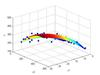linear regression

• ## 线性回归

万次阅读 多人点赞 2016-06-03 10:22:43
线性回归模型的基本特性就是：模型是参数的线性函数。最简单的线性回归模型当然是模型是参数的线性函数的同时，也是输入变量的线性函数，或者叫做线性组合。如果我们想要获得更为强大的线性模型，
线性回归（Linear Regression）

标签 : 监督学习

@author : duanxxnj@163.com
@time : 2016-06-19

对于一个的拥有m$m$个观测的训练数据集X$X$而言，回归的目的就是要对新的n$n$维输入x$x$，预测其对应的一个或者多个目标输出t$t$。

线性回归模型的基本特性就是：模型是参数的线性函数。

最简单的线性回归模型当然是模型是参数的线性函数的同时，也是输入变量的线性函数，或者叫做线性组合。

如果我们想要获得更为强大的线性模型，可以通过使用一些输入向量x$x$的基函数f(x)$f(x)$的线性组合来构建一个线性模型。这种模型，由于它是参数的线性函数，所以其数学分析相对较为简单，同时可以是输入变量的非线性函数。

从概率的角度来说，回归模型就是估计一个条件概率分布：p(t|x)$p(t|x)$。因为这个分布可以反映出模型对每一个预测值t$t$关于对应的x$x$的不确定性。基于这个条件概率分布对输入x$x$估计其对应的t$t$的过程，就是最小化损失函数（loss function）的期望的过程。

对于线性模型而言，一般所选择的损失函数是平方损失。

由于模型是线性的，所以在模式识别和机器学习的实际应用中存在非常大的局限性，特别是当输入向量的维度特别高的时候，其局限性就更为明显。但同时，线性模型在数学分析上相对较为简单，进而成为了很多其他的复杂算法的基础。

一般线性回归

对于一个一般的线性模型而言，其目标就是要建立输入变量和输出变量之间的回归模型。该模型是既是参数的线性组合，同时也是输入变量的线性组合。从数学上来说，如果定义y^$\hat{y}$为模型期望的输出，那么
y^(w,x)=w0+w1∗x1+w2∗x2+...+wn∗xn\hat{y}(w,x)= w_{0} + w_{1}*x_{1}+ w_{2}*x_{2}+...+ w_{n}*x_{n}

这里，我们定义ω={w0,w1,w2,...,wn}$\omega=\{w_{0},w_{1},w_{2},...,w_{n}\}$为参数向量， 若取x0=1$x_{0}=1$，则输入向量x={x0,x1,x2,...,xn}$x=\{x_{0},x_{1},x_{2},...,x_{n}\}$是n+1$n+1$维的。

在回归模型中，有一个比较特殊的回归模型：  逻辑回归(Logistic Regression)，这是一个用于分类的线性回归模型。

下面给出一份简单的线性回归的代码

#!/usr/bin/python
# -*- coding: utf-8 -*-

"""
author ： duanxxnj@163.com
time : 2016-06-19-20-48

这个是线性回归的示例代码
使用一条直线对一个二维数据拟合

"""
print(__doc__)

import matplotlib.pyplot as plt
import numpy as np
from sklearn import datasets, linear_model

# 加载用于回归模型的数据集
# 这个数据集中一共有442个样本，特征向量维度为10
# 特征向量每个变量为实数，变化范围（-.2 ，.2）
# 目标输出为实数，变化范围 （25 ，346）

# 查看数据集的基本信息
print diabetes.data.shape
print diabetes.data.dtype
print diabetes.target.shape
print diabetes.target.dtype

# 为了便于画图显示
# 仅仅使用一维数据作为训练用的X
# 这里使用np.newaxis的目的是让行向量变成列向量
# 这样diabetes_X每一项都代表一个样本
diabetes_X = diabetes.data[:, np.newaxis, 2]

# 此时diabetes_X的shape是(442L, 1L)
# 如果上面一行代码是：diabetes_X = diabetes.data[:, 2]
# 则diabetes_X的shape是(442L，)，是一个行向量
print diabetes_X.shape

# 人工将输入数据划分为训练集和测试集
# 前400个样本作为训练用，后20个样本作为测试用
diabetes_X_train = diabetes_X[:-20]
diabetes_X_test = diabetes_X[-20:]
diabetes_y_train = diabetes.target[:-20]
diabetes_y_test = diabetes.target[-20:]

# 初始化一个线性回归模型
regr = linear_model.LinearRegression()

# 基于训练数据，对线性回归模型进行训练
regr.fit(diabetes_X_train, diabetes_y_train)

# 模型的参数
print '模型参数：', regr.coef_
print '模型截距：', regr.intercept_

# 模型在测试集上的均方差（mean square error）
print("测试集上的均方差: %.2f"
% np.mean((regr.predict(diabetes_X_test) - diabetes_y_test) ** 2))
# 模型在测试集上的得分，得分结果在0到1之间，数值越大，说明模型越好
print('模型得分: %.2f' % regr.score(diabetes_X_test, diabetes_y_test))

# 绘制模型在测试集上的效果
plt.scatter(diabetes_X_test, diabetes_y_test,  color='black')
plt.plot(diabetes_X_test, regr.predict(diabetes_X_test), color='blue',
linewidth=3)

plt.grid()
plt.show()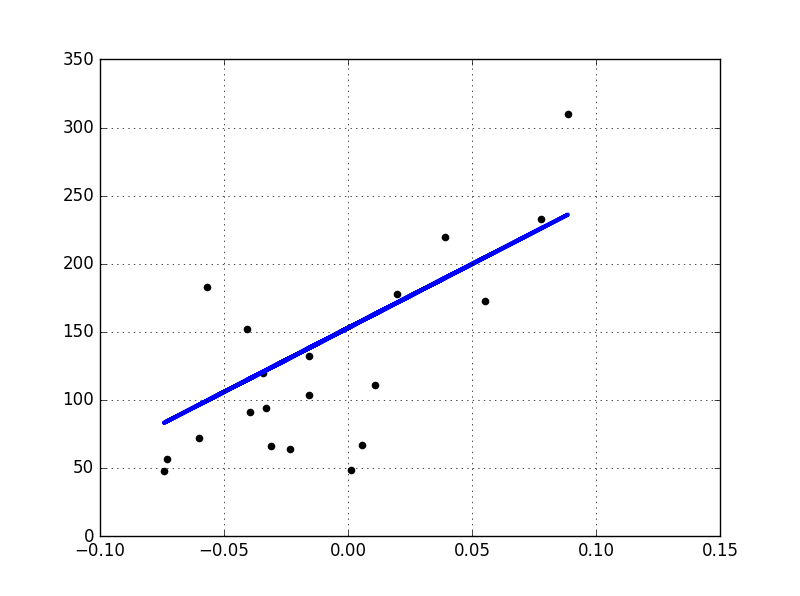最小二乘法（Ordinary Least Squares）

线性回归（Linear Regression）通过寻找合适的ω={w0,w1,w2,...,wn}$\omega=\{w_{0},w_{1},w_{2},...,w_{n}\}$，使得观测样本集合X$X$（这里观测样本集合X$X$在很多的外文书籍中比如RPML，叫做设计矩阵（design matrix）），和目标输出y$y$之间的残差平方和最小(residual sum of squares)，从数学上来说，其的目标问题或者叫做损失函数是：
J(w)=minw∥Xw−y∥2J(w)=\min_{w}\left \| Xw-y \right \|^{2}

最小乘法的证明

对于上面的损失函数而言，J(w)$J(w)$显然是非负的，其去最小值的充要条件就是∥Xw−y∥2$\left \| Xw-y \right \|^{2}$的倒数为0时的w$w$的取值，从矩阵的角度来写这个式子：
∥Xw−y∥2=(Xw−y)T(Xw−y)\left \| Xw-y \right \|^{2}=(Xw-y)^T(Xw-y)

容易得到其关于w$w$的导数为：
XT(Xw−y)+XT(Xw−y)=0X^T(Xw-y) + X^T(Xw-y)=0

即：
w=(XTX)−1XTyw=(X^TX)^{-1}X^Ty

这里，我们将X+≡(XTX)−1XT$X^+\equiv(X^TX)^{-1}X^T$定义为X的伪逆矩阵。

这个是一个非常好的结果，它说明使用最小二乘法，从数学上是可以直接通过公式来求解出参数w$w$的。

注意：最小二乘法对ω$\omega$的估计，是基于模型中变量之间相互独立的基本假设的，即输入向量x$x$中的任意两项xi$x_{i}$和xj$x_{j}$之间是相互独立的。如果输入矩阵X$X$中存在线性相关或者近似线性相关的列，那么输入矩阵X$X$就会变成或者近似变成奇异矩阵（singular matrix）。这是一种病态矩阵，矩阵中任何一个元素发生一点变动，整个矩阵的行列式的值和逆矩阵都会发生巨大变化。这将导致最小二乘法对观测数据的随机误差极为敏感，进而使得最后的线性模型产生非常大的方差，这个在数学上称为多重共线性（multicollinearity）。在实际数据中，变量之间的多重共线性是一个非常普遍的现象，其产生机理及相关解决方案在“特征选择和评估”中有介绍。

基于基函数的线性回归模型

前面已经提到过，线性回归模型的基本特征就是，模型是参数向量ω={w0,w1,w2,...,wn}$\omega=\{w_{0},w_{1},w_{2},...,w_{n}\}$的线性函数，上面的那个例子模型同时也是输入变量x$x$的线性函数，这极大地限制了模型的适用性。这里使用基函数（basis function）对上面的线性模型进行拓展，即：线性回归模型是一组输入变量x$x$的非线性基函数的线性组合，在数学上其形式如下：
y(x,w)=w0+∑j=1Mωjϕj(x)y(x,w)=w_{0}+\sum_{j=1}^{M}\omega_{j}\phi_{j}(x)

这里ϕj(x)$\phi_{j}(x)$就是前面提到的基函数，总共的基函数的数目为M$M$个，如果定义ϕ0(x)=1$\phi_{0}(x)=1$的话，那个上面的式子就可以简单的表示为：
y(x,w)=∑j=0Mωjϕj(x)=wTϕ(x)y(x,w)=\sum_{j=0}^{M}\omega_{j}\phi_{j}(x)=w^T\phi(x)

w=(w0,w1,w2,...,wM)w=(w_{0},w_{1},w_{2},...,w_{M})

ϕ=(ϕ0,ϕ1,ϕ2,...,ϕM)\phi=(\phi_{0},\phi_{1},\phi_{2},...,\phi_{M})

在“特征选择和评估”中，实际拿到手的数据，由于各种原因，往往不能直接应用到机器学习算法中，需要对原始数据做一些预处理或者是特征提取的操作，而那些从原始输入向量x$x$中提取出来的特征， 就是这一个个的ϕj(x)$\phi_{j}(x)$.

通过使用非线性的基函数，我们可以使得线性模型y(x,w)$y(x,w)$成为输入向量x$x$的非线性函数。

下面举一些基于不同基函数的线性模型y(x,w)$y(x,w)$的例子:

ϕj(x)=xj$\phi_{j}(x)=x_{j}$
这里取M=n$M=n$,那么就可以很容易的得到：
y(x,w)=w0+∑j=1Mωjϕj(x)=w0+w1∗x1+w2∗x2+...+wn∗xn
\begin{aligned}
y(x,w) &= w_{0}+\sum_{j=1}^{M}\omega_{j}\phi_{j}(x)\\
&=w_{0} + w_{1}*x_{1}+ w_{2}*x_{2}+...+ w_{n}*x_{n}
\end{aligned}

ϕj(x)=xj$\phi_{j}(x)=x^{j}$
这里就是典型的多项式曲线拟合（Polynomial Curve Fitting），其中M$M$是输入变量x$x$的最高次数，同时也是模型的复杂度。多项式曲线拟合的一个缺陷就是这个模型是一个输入变量的全局函数，这使得对输入空间的一个区间的改动会影响到其他的区间，这个问题可以通过将输入空间切分成不同的区域，对不同的区域各自在各自的区域中做曲线拟合来解决，这个方法就是经典的样条函数（spline function）。笔记中有专门的一节详细的论述了多项式曲线拟合相关的特性。
y(x,w)=w0+∑j=1Mωjϕj(x)=∑j=0Mωjxj
\begin{aligned}
y(x,w) &= w_{0}+\sum_{j=1}^{M}\omega_{j}\phi_{j}(x)\\
&= \sum_{j=0}^M\omega_{j}x^j
\end{aligned}

ϕj(x)=exp{−(x−μj)22s2}$\phi_{j}(x)=exp\{-\frac{(x-\mu_{j})^2}{2s^2}\}$
这里μj$\mu_{j}$表示的是该基函数在输入空间中的位置，参数s$s$控制的是基函数的空间尺度，这个基函数往往会被称为高斯基函数（Gaussian basis function）。需要强调一点，这里的高斯函数并不是输入空间的概率分布，其并没有概率意义。由于每个基函数前面都有一个比例系数ωj$\omega_{j}$，所以，对参数的归一化（coefficient normalization）并不是必需的。
y(x,w)=w0+∑j=1Mωjϕj(x)=w0+∑j=1Mωjexp{−(x−μj)22s2}
\begin{aligned}
y(x,w) &= w_{0}+\sum_{j=1}^{M}\omega_{j}\phi_{j}(x)\\
&=w_{0} + \sum_{j=1}^{M}\omega_{j}exp\{-\frac{(x-\mu_{j})^2}{2s^2}\}
\end{aligned}

ϕj(x)=σ(x−μjs)$\phi_{j}(x)=\sigma(\frac{x-\mu_j}{s})$
这个基函数叫做sigmoid$sigmoid$函数，其定义为：
σ(a)=11+exp(−a)\sigma(a)=\frac{1}{1+exp(-a)}
与这个函数拥有相同效果的一个函数叫做tach$tach$函数，其定义为：
tanh(a)=2σ(a)−1tanh(a)=2\sigma(a)-1

在神经网络中，这两个基函数有着非常广泛的应用。其实从根本上来讲，神经网络的每一层，也是一些基函数的线性组合，神经网络之所以能处理非线性问题，其根本也是由于采用了合适的非线性基函数。

y(x,w)=w0+∑j=1Mωjϕj(x)=w0+∑j=1Mωj11+exp(−a)
\begin{aligned}
y(x,w) &= w_{0}+\sum_{j=1}^{M}\omega_{j}\phi_{j}(x)\\
&=w_{0} + \sum_{j=1}^{M}\omega_{j}\frac{1}{1+exp(-a)}
\end{aligned}

线性回归的在线学习算法

在《多项式回归》中，介绍了线性模型的极大似然估计解释：对于训练数据{X,t}$\{X,t\}$而言，可以使用极大似然估计来计算参数w$w$和σ2$\sigma^2$，其中 w$w$ 是 y(xn,w)$y(x_{n},w)$ 的参数；而 σ2$\sigma^2$ 是噪声的方差：

p(t|X,w,σ2)=∏n=1NN(tn|y(xn,w),σ2)p(t|X,w,\sigma^2)=\prod_{n=1}^NN(t_{n}|y(x_{n},w),\sigma^2)

像OLS算法这种，一次性就将训练数据集中的N$N$个数据全部都用于p(t|X,w,σ2)$p(t|X,w,\sigma^2)$的估计中，然后通过求导得到参数的估计结果。这种算法的模型，称为批处理技术（Batch techniques），在Andrew Ng的机器学习课程视频中，对这个有说明。其主要缺点就在于，面对大的数据集的时候，其计算成本会非常的大。

当数据集特别大的时候，这种批处理技术就不再适用，就有一种称为序列学习算法（Sequential learning），也叫作在线算法（on-line algorithms）的算法得到了广泛的应用。

说得简单一点，序列学习算法就是将数据的样本点，看成在时间上有序的，即，数据集合不是一次性得到全部的数据，而是一个样本一个样本采集得到的。那么，在训练算法的时候，也就可以一个样本一个样本的输入的训练算法的中，利用新的样本不断的改进模型，进而达到学习的目的。

梯度下降法

有前面可以知道，线性回归的损失函数为:

J(w)=minw∥Xw−y∥2J(w)=\min_{w}\left \| Xw-y \right \|^{2}

其关于参数w$w$梯度为：

∇J(w)=2XT(Xw−y)\nabla J(w)=2X^T(Xw-y)

梯度下降法就是：

wk=wk−1−ρk∇J(w)w_{k} = w_{k-1}- \rho_{k} \nabla J(w)

通过迭代，求得wk$w_{k}$

随机梯度下降法

随机梯度下降法，就是这里要说的在线学习算法，它不像上面的梯度下降法，每次迭代都使用了全部的数据集，这里每次迭代仅仅使用一个样本：

wk=wk−1−ρkxk(xTkwk−1−yk)w_{k} = w_{k-1}- \rho_{k} x_{k}(x_{k}^Tw_{k-1} - y_{k})

这里需要说明的是，ρk$\rho_{k}$ 必须满足两个条件：

∑k=1∞ρk→∞∑k=1∞ρ2k<∞\sum_{k=1}^{\infty}\rho_{k}\to\infty \\
\sum_{k=1}^{\infty}\rho_{k}^2 < \infty

这个过程，其实是根据新的输入样本，对wk$w_{k}$的一个不断的修正的过程，一般来说，我们会把ρk$\rho_{k}$后面的式子称为修正量。

折两个条件，保证了在迭代过程中估计的修正值，会趋近于零。因此，对于足够大的k$k$而言，迭代会突然停止，但是，由于有第一个条件的存在，这个停止不会发生的太早，并且不会再离结果非常远的时候，就停止了迭代。上面的第二个条件保证了，对于变量的随机性噪声，噪声的累积保持有限。
展开全文• 现在用 Python 写线性回归的博客都快烂大街了，为什么还要用 SPSS 做线性回归呢？这就来说说 SPSS 存在的原因吧。 SPSS 是一个很强大的软件，不用编程，不用调参，点巴两下就出结果了，而且出来的大多是你想要的。...
现在用 Python 写线性回归的博客都快烂大街了，为什么还要用 SPSS 做线性回归呢？这就来说说 SPSS 存在的原因吧。

SPSS 是一个很强大的软件，不用编程，不用调参，点巴两下就出结果了，而且出来的大多是你想要的。这样的特点特别适合建模初期进行算法的选择。比如

SPSS 做因子分析，输出结果中有一项 Kaiser-Meyer-Olkin Measure of Sampling Adequacy. 它的值是在 [ 0, 1] 范围内，这个值大于 0.5 就证明原数据中的指标适合使用因子分析算法进行建模，小于 0.5 要不重新计算指标，要不换算法。
SPSS 做多元线性回归，输出结果中的拟合度过低，说明指标与结果之间的相关性并不明显，要不重新计算指标，要不换算法。
..................
下面详细讲讲 SPSS做多元线性回归的步骤吧

准备工作：SPSS - 中文版 SPSS 22.0 软件下载与安装教程 - 【附产品授权许可码，永久免费】

第一步：导入数据

路径：【文件】--【打开】--【数据】--【更改文件类型，找到你的数据】--【打开】--【然后会蹦出下图左中的筛选框，基本使用默认值就行，点确定】

第一行代表的是用第一行的数据做列名；
第二行代表文件中数据所在的范围，默认是所有数据都选上，但是如果你只需要选择前 n 行，那就把里面的110改了就行；
第三行代表字符串宽度，这个默认值就可以，不用改；
导入数据之后就是下图右中的样子，老习惯，我们来说说原数据，第一列是拨打电话指数，第二列是接通电话指数，这两个是自变量，第三类是因变量回款指数。为了脱敏，所以用自己的办法换算成现在这样的数值。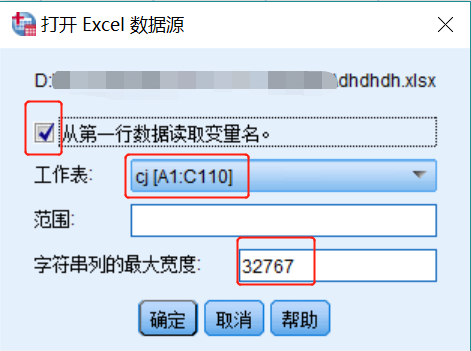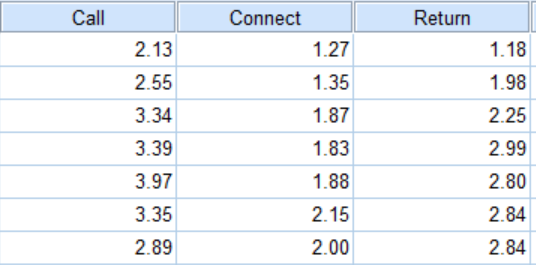第二步：数据分析

【分析】--【回归】--【线性】--【通过截图中的方式，将因变量与自变量添加到对应的地方】--【其他都使用默认值】--【确定】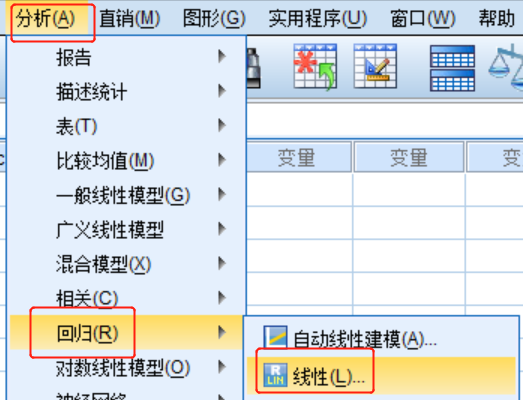第三步：输出结果分析

第一项输出结果：输入／移去的变量

输入变量是两个自变量Connect, Call，没有移去任何变量。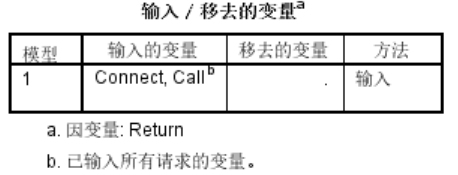第二项输出结果：模型汇总

R表示拟合优度（goodness of fit），用来衡量模型的拟合程度，越接近 1 越好；
R方表示决定系数，用于反映模型能够解释的方差占因变量方差的百分比，越接近 1 越好；
调整R方是考虑自变量之间的相互影响之后，对决定系数R方的校正，比R方更加严谨，越接近 1 越好；
标准估计的误差是误差项 ε 的方差 σ2的一个估计值，越小越好；
一般认为，

小效应：R （0.1~0.3），对应 R方（0.01~0.09）;
中等效应：R （0.3~0.5），对应 R方（0.09~0.25）;
大效应：R （0.5~1），对应 R方（0.25~1）;第三项输出结果：Anova

Anova表示方差分析结果，主要看 F 和 Sig 值，为方差分析的结果，F检验的重点在 Sig 值，具体大小不重要，其 F 值对应的 Sig 值小于 0.05 就可以认为回归方程是有用的。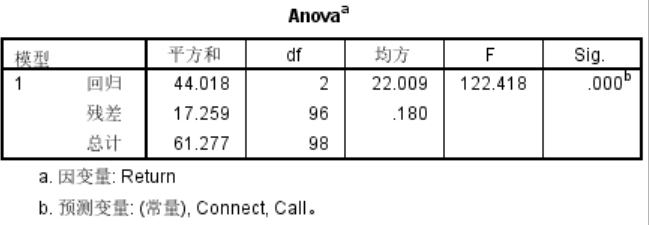第四项输出结果：系数

系数表列出了自变量的显著性检验结果，

非标准化系数中的 B 表示自变量的系数与常数项（下图代表的回归方式为：Return = 0.097 * Call + 1.243 * Connect - 0.160）；
标准系数给出的自变量系数与非标准化系数中的明显不同，这是因为考虑到不同自变量之间的量纲和取值范围不同（比如在其他例子里面，第一个自变量是年龄（0~120），第二个自变量是收入（0~10万），显然年龄18岁与收入18块钱代表的意义是不一样的，因此需要进行标准化），因此这里的系数更能代表每个自变量对因变量的影响程度，（下图代表的回归方式为：Return = 0.126 * Call_标准化的值 + 0.739 * Connect_标准化的值）；
t 值 与 Sig 值 是自变量的显著性检验结果，其 t 值对应的 Sig 值小于 0.05 代表自变量对因变量具有显著影响，下图中，自变量 Connect 对 因变量具有显著影响，而自变量 Call 的影响程度就弱了很多；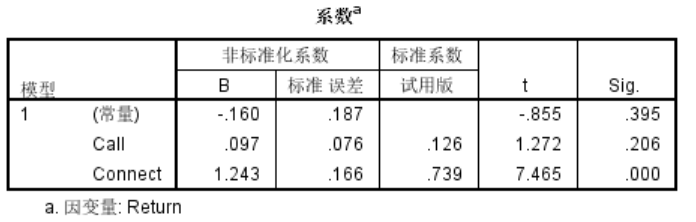综上所有的输出结果，说明 Call、 Connect 与 Return 的拟合效果还挺理想的。

与Python的结果对比

同样的数据，我们看看Python中的多元线性回归结果：

Python给出的回归方程： Y = -0.01 + 0.09 * Call + 1.19 * Connect；

SPSS 给出的回归方程： Y = -0.16+ 0.09 * Call + 1.24 * Connect；

如果想要学习一下这个过程，你可能需要：

1.SPSS - 中文版 SPSS 22.0 软件下载与安装教程 - 【附产品授权许可码，永久免费】

2.机器学习 - 多元线性回归 - 一步一步详解 - Python代码实现

本例中用的数据与机器学习 - 多元线性回归 - 一步一步详解 - Python代码实现中的数据是同一份，便于对比。
展开全文数据建模 Python
• 最近做了一些用SPSS进行线性回归的实验，还是感觉很多细节把握不好，这里结合我的实验结果，以及网上别人的介绍总结一下，先贴几张SPSS的输出： 下面简单解释一下这三张图中的结果： 第一个表模型汇总表中，...
先说一句题外话，如果当年在大学里数理统计等课程结合SPSS，SAS，R等软件来讲，应该效果会好很多。

最近做了一些用SPSS进行线性回归的实验，还是感觉很多细节把握不好，这里结合我的实验结果，以及网上别人的介绍总结一下，先贴几张SPSS的输出：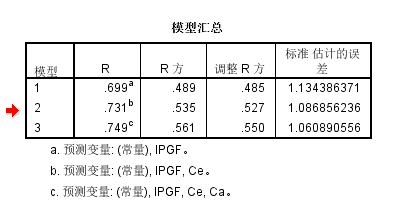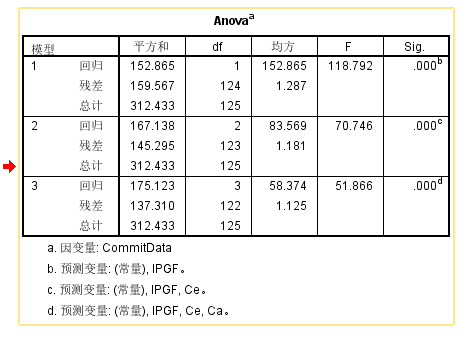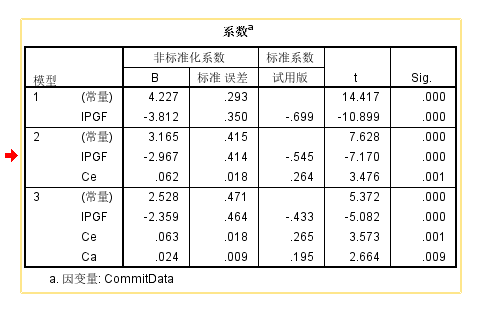下面简单解释一下这三张图中的结果：

第一个表模型汇总表中，R表示拟合优度（goodness of fit），它是用来衡量估计的模型对观测值的拟合程度。它的值越接近1说明模型越好。调整的R平方比调整前R平方更准确一些，图中的最终调整R方为0.550，表示自变量一共可以解释因变量55%的变化（variance），另外，由于使用的是StepWise Linear Regression (SWLR)，分析——回归——线性——“方法”选择“逐步”，所以模型1、2、3的R方逐渐增大，标准误差逐渐减小。

（据网友的介绍：一般认为，拟合优度达到0.1为小效应（R方0.01），0.3为中等（R方0.09），0.5为大（R方0.25），这是针对自然科学的一般界限。）

第二个表Anova表示方差分析结果，主要看F和sig值两个，F值为方差分析的结果，是一个对整个回归方程的总体检验，指的是整个回归方程有没有使用价值（与随机瞎猜相比），其F值对应的Sig值小于0.05就可以认为回归方程是有用的。另外，从F值的角度来讲：F的值是回归方程的显著性检验，表示的是模型中被解释变量与所有解释变量之间的线性关系在总体上是否显著做出推断。若F>Fa(k,n-k-1)，则拒绝原假设，即认为列入模型的各个解释变量联合起来对被解释变量有显著影响，反之，则无显著影响。

这里简单对Fa(k,n-k-1)进行一下解释，k为自变量个数，n为样本容量，n-k-1为自由度。对于我的实验中的情况来讲，k=3，样本容量为146，所以查表的时候应该差Fa(3,142)，一般数理统计课本中都有F分布表，a表示的显著性水平（一般取0.05），但我们手头不一定会有课本，就需要借助于excel来查F表，打开excel，在公式区输入：=FINV(0.05,3,142)，在单元格中即出现2.668336761，表中的F值显著大于这个值，则认为各个解释变量对因变量有显著影响。

需要注意的是，方差分析是对多个自变量的总体检验，而不是单个自变量（单个自变量在系数表中，为单样本T检验），这就是第三个表回归系数表中的内容。

系数表格列出了自变量的显著性检验结果（使用单样本T检验），最后一列为T检验的sig，表中均小于0.05，说明自变量对因变量具有显著影响，B表示各个自变量在回归方程中的系数，负值表示IPGF这个自变量对因变量有显著的负向影响，但是由于每个自变量的量纲和取值范围不同，基于B并不能反映各个自变量对因变量影响程度的大小，这时候我们就要借助标准系数。目前表格中的“试用版”实际上是Beta的意思，此时数值越大表示对自变量的影响更大。

从这个分析过程来看，这个实验结果还挺理想的。

展开全文• ## stata 线性回归分析基本操作

万次阅读 多人点赞 2019-09-18 09:55:53
一、线性回归基本命令 regress y x1 x2 （红色表示该命令可简写为红色部分） 以 Nerlove 数据为例（数据附后文） regress lntc lnq lnpf lnpk lnpl 表上半部分为方差分析表，包括回归平方和，残差平方和，...
一、线性回归基本命令

regress y x1 x2   （红色表示该命令可简写为红色部分）

以 Nerlove 数据为例（数据附后文）

regress lntc lnq lnpf lnpk lnpl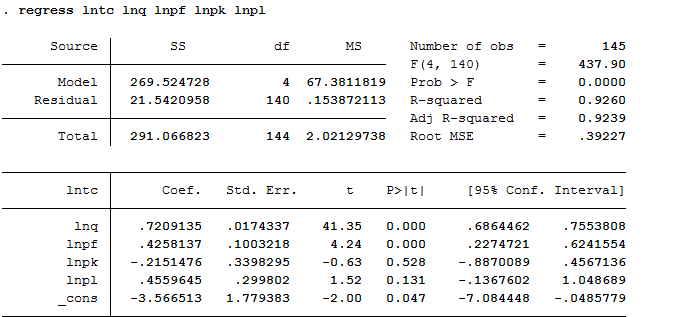表上半部分为方差分析表，包括回归平方和，残差平方和，均方，F检验等。上半部分右侧给出拟合优度R2和调整的R2。root MSE 表示方程的标准误差。

表下半部分为回归系数的点估计和区间估计值，标准误和t检验值。

如果回归不需要常数项，则可在命令后面加上 noconstant

regress lntc lnq lnpf lnpk lnpl,noc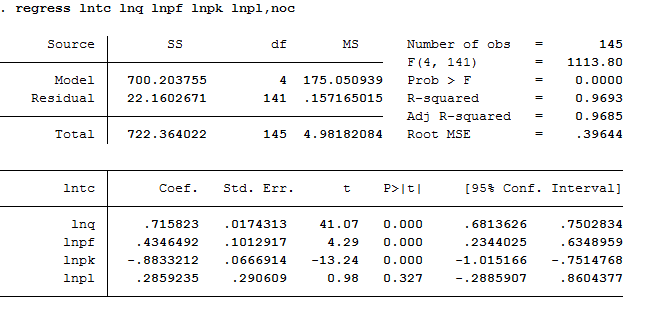二、带约束条件的回归

如果只对满足一定条件的样本进行回归，可增加条件项  if 条件

假如本例中q>=6000认定为大企业，则可设置如下命令

 regress lntc lnq lnpf lnpk lnpl if q>=6000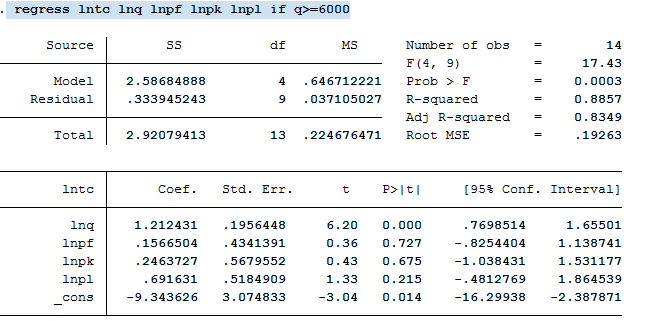或者使用虚拟变量表示，定义一个新变量large ,如果是大企业，则取值为1，否则为0，代码为

 g large=(q>=6000)

 regress lntc lnq lnpf lnpk lnpl if large

输出结果等价于上面if条件结果。

如果对非大企业进行回归，则可表示为

 regress lntc lnq lnpf lnpk lnpl if large==0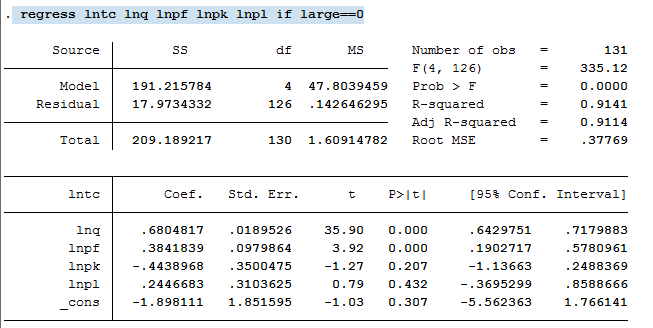如果回归系数需满足某些指定条件，比如a1+a2+a3=1,a1=2a2 等，则可通过设置约束条件完成：

 constraint def 1 lnpl+ lnpk+ lnpf=1

cnsreg lntc lnq lnpf lnpk lnpl,c(1)

constraint def  1  定义第一个约束条件，cnsreg表示带约束回归，c(1) 表示满足约束条件1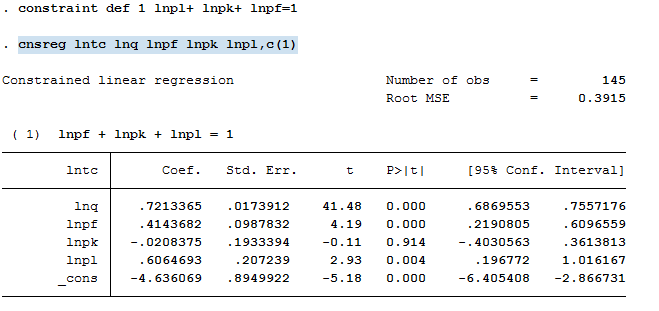如果需要同时满足几个条件，可以进一步设置条件2，条件3，以新增约束条件lnq=1 为例

 cons def 2 lnq=1

cnsreg lntc lnq lnpf lnpk lnpl,c(1-2)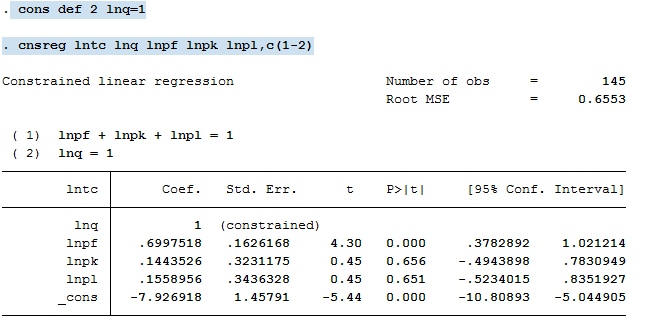三、预测、检验和相关计算

如果要计算因变量的拟合值，并保存到新变量yhat中，则可采用predict：

以对无约束条件的回归进行预测为例：

regress lntc lnq lnpf lnpk lnpl

predict yhat

预测结果保存在原始数据集中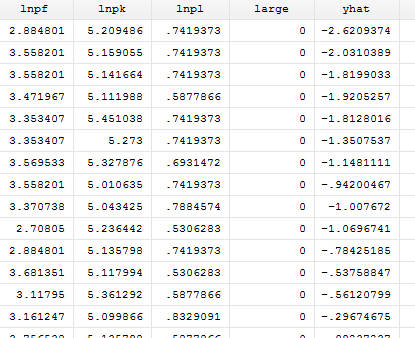进一步计算残差，并保存到e1中，则可（其中residual可简写）：

 predict e1,residual

残差e1结果保存在原始数据中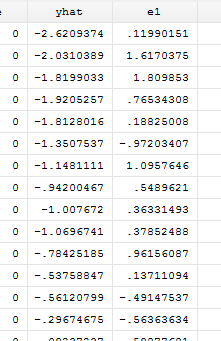如果要对某个系数进行计算，可直接采用display 表达式方式，比如计算lnq的平方

 di _b[lnq]^2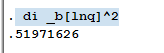如果需要对系数进行某种条件检验，可采用test 条件，以检验lnq=1，lnpl+lnpk+lnpf=1 为例

 te lnq=1
te (lnq=1)(lnpl+lnpk+lnpf=1)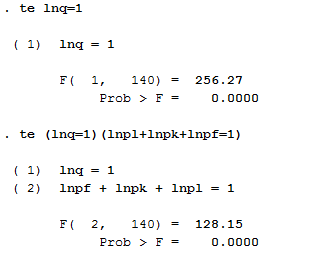单独检验lnq=1，F检验显示拒绝原假设；联合检验lnq=1，lnpl+lnpk+lnpf=1，拒绝联合成立的原假设。

对两个系数同时等于0进行假设，可采用命令 te 变量1 变量2 ，以检验lnpl lnpk联合等于0为例：

 test lnpl lnpk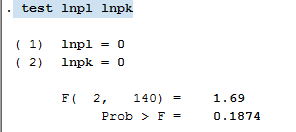F检验表明不拒绝两者皆为0的原假设。

展开全文• ## spss进行多元线性回归并分析表格

万次阅读 多人点赞 2019-04-01 00:50:26
1.如何使用spss进行多元线性回归。 2.分析生成结果，并判断回归是否可行。 一、使用spss进行多元线性回归： 1.输入数据 2. 3. 4. 5. 6. 二、表格结果分析： R方是检验回归是否成功的重要要素...
• 本课程主要讲述如何使用python进行线性回归与非线性回归分析，包括： 基于statsmodel的线性回归方法 基于sklearn的线性回归方法 基于Numpy的一元多项式非线性回归方法 ...大数据 大数据技术 大数据平台 大数据技术原理与应用 大数据学习路线
• 一、多元线性回归 所谓的多元线性回归就是指在回归分析中，如果有两个或两个以上的自变量，就称为多元回归。 二、多元线性回归模型 1.建立模型 以二元线性回归模型为例 ，二元线性回归模型如下： 类似的使用最小...
• 当影响因变量的因素是多个时候，这种一个变量同时与多个变量的回归问题就是多元回归，分为：多元线性回归和多元非线性回归。这里直说多元线性回归。对比一元线性回归： 1.1多元回归模型： y=β0+β1x1+β2x2+…+β...
• ## 多元线性回归模型

万次阅读 多人点赞 2019-07-02 19:22:12
多元线性回归模型通常用来研究一个应变量依赖多个自变量的变化关系，如果二者的以来关系可以用线性形式来刻画，则可以建立多元线性模型来进行分析。 1.模型简介 1.1模型的结构 多元线性回归模型通常用来描述变脸y和x...
• 回归分析是数据分析中最基础也是最重要的分析工具，绝大多数的数据分析问题，都可以使用回归的思想来解决。回归分析的任务就是， 通过研究自变量X和因变量Y的相关关系，尝试去解释Y的形成机制，进而达到通过X去预测Y...异方差检验 多重共线性 逐步回归
• ## 多元线性回归 stata

千次阅读 多人点赞 2019-09-16 09:43:18
回归的思想，任务，使命 可以看到0-1回归的因变量自变量都是0-1变量 因变量y可以分为 解释y的形成机制就是通过建立x，y的数学关系式 感觉相关分析就是回归分析的前两步， 数据的分类 ...
• 单变量线性回归 绘制散点图 相关系数R 拆分训练集和测试集 多变量线性回归 数据检验（判断是否可以做线性回归） 训练线性回归模型 先甩几个典型的线性回归的模型，帮助大家捡起那些年被忘记的数学。 ● 单...python 回归分析
• 回归分析中，如果有两个或两个以上的自变量，就称为多元回归。事实上，一种现象常常是与多个因素相联系的，由多个自变量的最优组合共同来预测或估计因变量，比只用一个自变量进行预测或估计更有效，更符合实际。 ...matlab
• 这一篇文章，我们将首次介绍回归算法即对连续性的数据做出预测。回归一词的来历由来已久。“回归”是由英国著名生物学家兼统计学家高尔顿(Francis Galton,1822～1911.生物学家达尔文的表弟)在研究人类遗传问题时提...lr lasso...